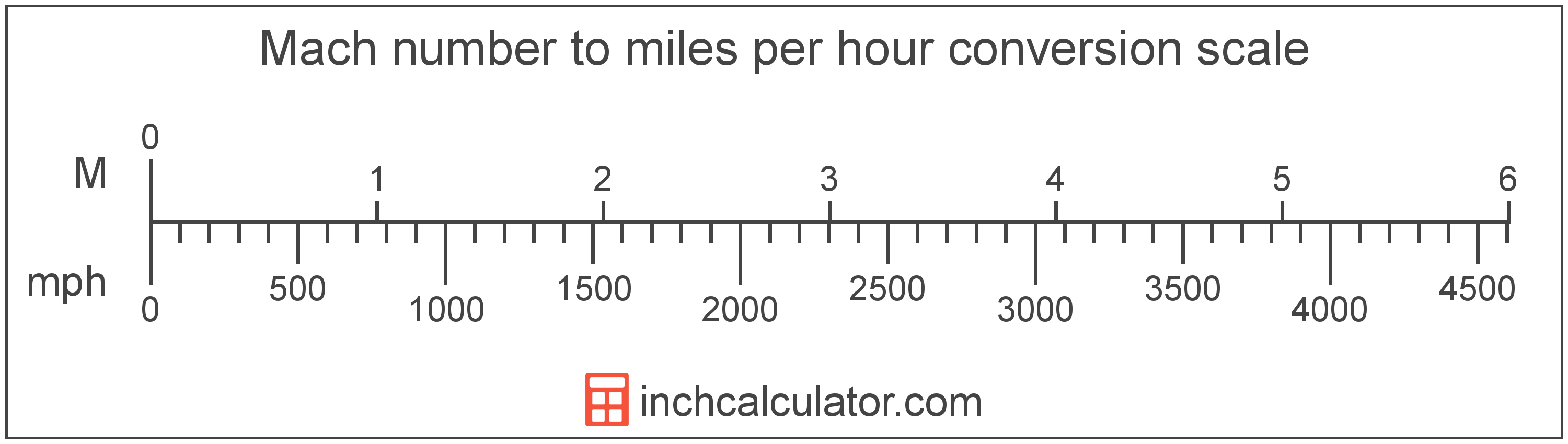# Mach Number to Miles per Hour Converter

Enter the Mach number below to convert the speed to miles per hour.

Results in Miles per Hour:Mach 1 = 767.269148 mph

Do you want to convert miles per hour to Mach number?

## How to Convert Mach Number to Miles per Hour

To convert a Mach measurement to a mile per hour measurement, multiply the Mach number by the conversion ratio. Mach 1 is equal to 767.269148 miles per hour, so use this simple formula to convert:

miles per hour = Mach number × 767.269148

The speed in miles per hour is equal to the Mach number multiplied by 767.269148.

For example, here's how to convert Mach 2 to miles per hour using the formula above.
Mach 2 = (2 × 767.269148) = 1,534.538296 mphMach number and miles per hour are both units used to measure speed. Keep reading to learn more about each unit of measure.

## What Is Mach Number?

Mach, sometimes also referred to as a Mach number, is the ratio of the speed of an object to the speed of sound.

Mach can be abbreviated as M, and is also sometimes abbreviated as Ma. For example, Mach 1 can be written as 1 M or 1 Ma.

Although Mach can be abbreviated M or Ma, the Mach number is typically expressed with the number after the unit name. For example, the expression Mach 3 is preferred rather than 3 Machs or 3 M.

The speed is classified as either subsonic, transonic, supersonic, or hypersonic depending on the Mach number.

Speed classifications for various mach numbers.
Speed Classification Mach Number
Subsonic Mach < 1.0
Transonic Mach = 1.0
Supersonic Mach > 1.0
Hypersonic Mach > 5.0

## What Are Miles per Hour?

Miles per hour are a measurement of speed expressing the distance traveled in miles in one hour.

The mile per hour is a US customary and imperial unit of speed. Miles per hour can be abbreviated as mph, and are also sometimes abbreviated as mi/h or MPH. For example, 1 mile per hour can be written as 1 mph, 1 mi/h, or 1 MPH.

Miles per hour can be expressed using the formula:
vmph = dmi / thr

The velocity in miles per hour is equal to the distance in miles divided by time in hours.

## Mach to Mile per Hour Conversion Table

Mach numbers converted to miles per hour
Mach Number Miles Per Hour
Mach 0.25 191.82 mph
Mach 0.5 383.63 mph
Mach 0.75 575.45 mph
Mach 1 767.27 mph
Mach 1.5 1,151 mph
Mach 2 1,535 mph
Mach 2.5 1,918 mph
Mach 3 2,302 mph
Mach 3.5 2,685 mph
Mach 4 3,069 mph
Mach 4.5 3,453 mph
Mach 5 3,836 mph
Mach 5.5 4,220 mph
Mach 6 4,604 mph
Mach 6.5 4,987 mph
Mach 7 5,371 mph
Mach 7.5 5,755 mph
Mach 8 6,138 mph
Mach 8.5 6,522 mph
Mach 9 6,905 mph
Mach 9.5 7,289 mph
Mach 10 7,673 mph

## References

1. NASA, Mach Number, https://www.grc.nasa.gov/WWW/BGH/mach.html
2. Wikipedia, Miles per hour, https://en.wikipedia.org/wiki/Miles_per_hour